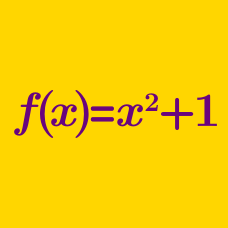Algebra

# Functions: Level 4 Challenges

Andrew has a favorite function $A(x)=px+q^x$ such that $A(1)=4$ and $4A(2)=37$, find the maximum value of $p-q.$

###### Try Part 1.

Suppose $f$ is a continuous, positive real-valued function such that $f(x + y) = f(x)f(y)$ for all real $x,y.$

If $f(8) = 3$ then $\log_{9}(f(2015)) = \dfrac{a}{b}$, where $a$ and $b$ are positive coprime integers. Find $a - b.$

\begin{aligned} e(x)+o(x) &= & f(x) \\ e(x)+x^2& =& o(x) \\ \end{aligned} If the above two equations are true for every real $x$ where $e(x)$ and $o(x)$ are any even and odd functions respectively whereas $f(x)$ may be an ordinary function. Find $f(2).$

The lovely function $f_{}^{}$ has the beautiful property that, for each real number $x$:

$\large f(x)+f(x-1) = x^2.$

If $f(19)=94$, what is $f(94)$?

###### This is original.

\begin{aligned} f_1 (x)&=&x\\ f_2 (x)&=&1-x\\ f_3 (x)&=&\frac{1}{x}\\ f_4 (x)&=&\frac{1}{1-x}\\ f_5 (x)&=&\frac{x}{x-1}\\ f_6 (x)&=&\frac{x-1}{x}\\ \end{aligned}

If we know that $f_6 (f_m(x))=f_4(x)$ and $f_n (f_4(x))=f_3(x)$, find the minimum value of $m+n$.

×